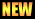# MTH322: Real Analysis II (Fall 2018)

This course is offered to MSc, Semester II at Department of Mathematics, COMSATS University Islamabad, Attock campus. This course need rigorous knowledge of continuity, differentiation, integration, sequences and series of numbers, that is many notion included in Real Analysis I.

Sequences of functions: convergence, uniform convergence, uniform convergence and continuity, uniform convergence and integration, uniform convergence and differentiation, the exponential and logarithmic function, the trigonometric functions.

Series of functions: Absolute convergence, uniform convergence, Cauchy criterion, Weiestrass M-test, power series of functions, radius of convergence, Cauchy-Hadamard theorem, differentiation theorem, uniqueness theorem.

Improper integrals: Improper integral of first and second kind, comparison tests, Cauchy condition for infinite integrals, absolute convergence, absolute convergence of improper integral, uniform convergence of improper integrals, Cauchy condition for uniform convergence, Weiestrass M-test for uniform convergence.

#### Assignment

• Assignment 01 (107.79 KiB, 55 downloads) | View Online#### Online resources

1. Brian S. Thomson, Judith B. Bruckner, and Andrew M. Bruckner, Elementary Real Analysis:Second Edition (2008) URL: http://classicalrealanalysis.info/Elementary-Real-Analysis.php
2. Rudin, W. (1976). Principle of Mathematical Analysis, McGraw Hills Inc.
3. Bartle, R.G., and D.R. Sherbert, (2011): Introduction to Real Analysis, 4th Edition, John Wiley & Sons, Inc.
4. Apostol, Tom M. (1974), Mathematical Analysis, Pearson; 2nd edition.
5. Somasundaram, D., and B. Choudhary, (2005) A First Course in Mathematical Analysis, Narosa Publishing House.
6. S.C. Malik and S. Arora, Mathematical analysis, New Age International, 1992. (Online google preview)
• atiq/fa18-mth322